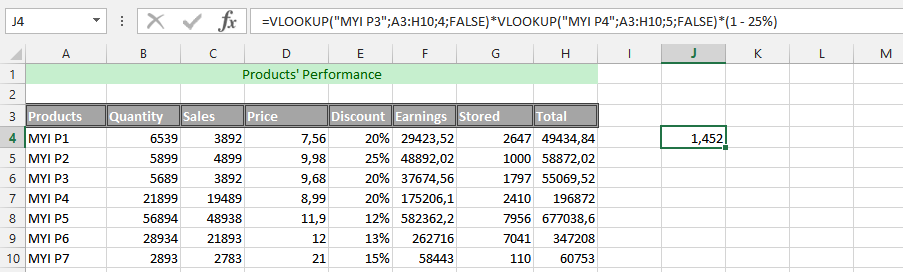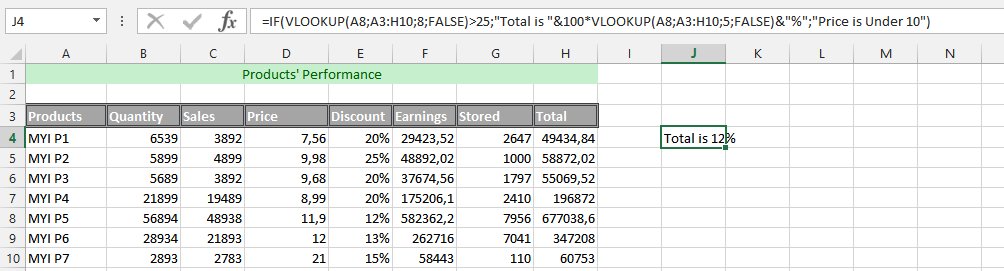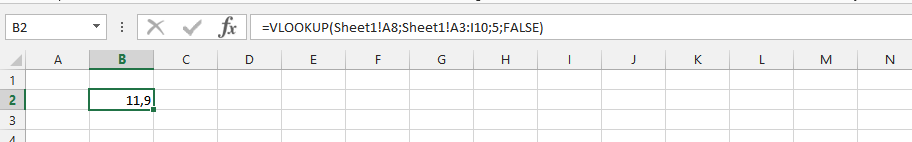### VLOOKUP Function: Knowing It & 10 Examples Of Its Usage

This is the function that would search for a specific value in first column that is in the table array, so it would be returning with a value that is in the same row, and placed in a different column that is in the same table array. V in the VLOOKUP means vertical, which makes it more beneficial than HLOOKUP when a comparing value is being located in a column that is on the left of a data that user wants to find.

Understanding VLOOKUP and its Parameters

The VLOOKUP has multiple parameters that works together in finding the value that the user is looking for with it. The function has a lookup value, table of array, index’s column number, and the range lookup.

Lookup Value: This is a value that user want to search in a first column of a table’s array. This can be either a reference or value. If there is a lookup value that is smaller than smallest value in the same first column of that table array, it would only leads to the VLOOKUP returning with the #N/A error value.

Table Array: This needs to be either two or more columns that contain data. It is possible for this to be either a range name or a range’s reference. Values that are in this array’s first column would be searched by a lookup array. These values can be logical values, numbers or even text. Both the upper- and lowercase texts are equal to the array.

Column Index Number: This is a column number that is in the table array, which is where a matching value is required to be matched. If it is 1, then it would return with a value that is in first column in that table’s array, if it is 2, then it would return with the second column in the array, 3 will return with the third column, and so on. If it is less than 1, then it will be returning with than error value that says #Value! However, if the number is being greater than number of columns in a table’s array, then the VLOOKUP function would be returning with the error value that says #REF!

Range Lookup: This is the logical value that would be specifying if the user wants the VLOOKUP function to either find the exact match of the value, or just make an approximating match.

### Example 1: Simple VLOOKUP Function

The example is going to make it possible for knowing if the goal that has been set has been achieved in this example. We’d have a product that needs to achieve a specific goal within a period of time, and now we want to know how the sales has been on the market. This is where the VLOOKUP formula is going to be extremely useful.### Example 2: Usage of VLOOKUP

Twice in the same Formula In this example, we are aiming to use the VLOOKUP formulas twice, and using them to know the net worth of the sales that has been made. It will makes it possible for finding a solid details that will get us the answer that we are looking after, with the combinations of the two formula. Let us use the same data as the one on top. This time, we have also added another data to the whole sheet, which is the total income we expects form selling the whole products. It is about knowing the performance of a specific product, comparing the total earnings with revenues.This example is using the very same data as the previous one, and this time we have also set a goal for the whole product. We have already set out how much the business would be going under the circumstances that our goal has been set, and we know that we want to set a specific percentage of the whole price away from the whole retail price. This discount is about making it possible for us to get rid of the discount that has been meant to be discounted from the retail price. For this to be successful, we need to add a new column to the data, which would be the price. This is where the formula would be very useful for getting the answer that we’d like to get. We want to know how the sales would be affected.### Example 4: Easy IF and VLOOKUP

This is an example, where we are using the IF formula with Vlookup formula at ease. We are using this to find out how the business is being affected by the decision, so we are using the IF and VLOOKUP formula to find the answer we needs. This is because we want to know how much the discount would be, when it has been subtracted from the total earnings.### Example 5: Double VLOOKUP Formula with IF Formula

This example is about finding out the percentage it would cost us under the circumstances that it would be very difficult for us to find out under another circumstances. The best way to do it is finding out what the value it would cost us with the discount that has been given to the products, due to the upcoming sales period, and we would want to know how this would have effect on the revenues coming into the business as a result. This can be something that would help in acknowledging the business process.### Example 6: Age of Sales with Four Different Formulas

In this example, we’d have different performances, and we want to know the age of our sales, and determine the age. This is where four different formulas are useful in the long run, and the circumstances would require having a pure understanding of simultaneously using the formulas. These formulas are INT, Yearfrac, Date and the Vlookup that work together to provide the result we desire.### Example 7: Simultaneously Using VLOOKUP Function with 2 other Functions

The example is about using the IF, ISNA and VLOOKUP formula at the same time. This is what we needs to use for finding out about the information that shows about if our aim has been achieved or not. The function has been based on finding a solid and appropriate information that makes it possible and easy to know the answer.### Example 8: Triple VLOOKUP Formula

This example is about using three different Vlookup formulas simultaneously, and finds answer that we are looking for, which would make it possible and easy to find a desired answers.### Example 9: VLOOKUP with Texts and Number

In this example, we are going to use the VLOOKUP formula with regards to all data being in text, and we are trying to use the VLOOKUP formula to find the value that we are looking for, and this is under the circumstances that the whole data has been given a label to make it successful.### Example 10: VLOOKUP from a Different Spreadsheet

This example is an excellent formula, under the circumstances that we have multiple years of experiences in the business, previous year has been put into a Microsoft Excel’s spreadsheet, while we have the current year in another spreadsheet, but in the same document. This is where the VLOOKUP formula needs to be used in regards to the two spreadsheets.## Template

```Further reading: